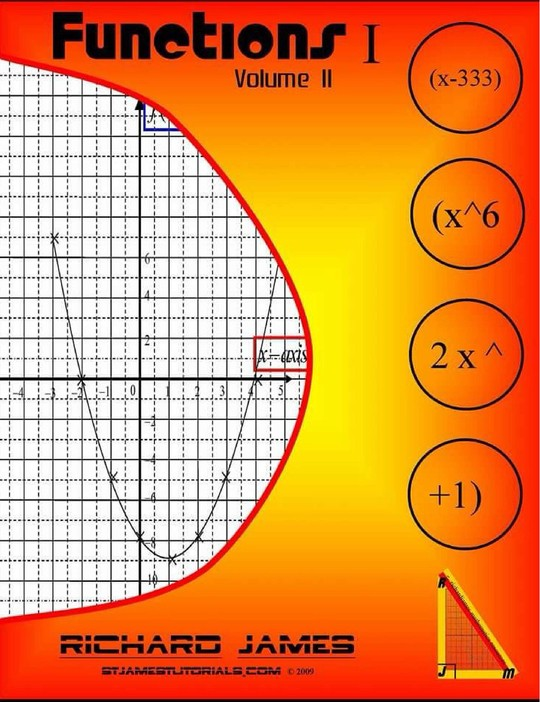\$15.63

# Volume 2: Functions I

By Richard James
US\$ 15.63
The publisher has enabled DRM protection, which means that you need to use the BookFusion iOS, Android or Web app to read this eBook. This eBook cannot be used outside of the BookFusion platform.
Book Description

Volume 2: Functions 1:- This branch of mathematics is so diverse that I have decided to divide it into two parts. This first section begins with the most basic form of a function - mapping and continues with a comprehensive view of the rectangular coordinate system. Earlier on, we have the manipulation of functions including evaluation, inverse and composition. We then move on to other algebraic and graphical representations for example quadratic expressions and graphs; straight line expressions and graphs and the gradient of a curve. The final topics in completing the square would also be very good introductory topics for the subsequent volume.

Table of Contents
• Cover Page
• 17 Functions
• Introduction
• 18 Quadratic Graphs
• Introduction
• 22 The Equation of a Straight Line
• Introduction
• 23 Straight Line Graphs
• Introduction
• 24 Gradient of a curve
• Introduction
• 25 Completing the Square
• Introduction
• 26 Solution of Quadratic Equation By Completing The Square
• Introduction
No comment for this book yet, be the first to comment
You May Also Like